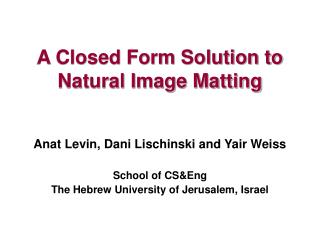DownloadDownload PresentationA Closed Form Solution to Natural Image Matting

# A Closed Form Solution to Natural Image Matting

Download Presentation## A Closed Form Solution to Natural Image Matting

- - - - - - - - - - - - - - - - - - - - - - - - - - - E N D - - - - - - - - - - - - - - - - - - - - - - - - - - -
##### Presentation Transcript

1. A Closed Form Solution to Natural Image Matting Anat Levin, Dani Lischinski and Yair Weiss School of CS&Eng The Hebrew University of Jerusalem, Israel

2. The matting equations = x + x

3. Why is matting hard?

4. Why is matting hard?

5. Why is matting hard?

6. Why is matting hard? Matting is ill posed: 7 unknowns but 3 constraints per pixel

7. Previous approaches • The trimap interface: • Bayesian Matting (Chuang et al, CVPR01) • Poisson Matting (Sun et al SIGGRAPH 04) • Random Walk (Grady et al 05) • Scribbles interface: • Wang&Cohen ICCV05

8. Problems with trimap based approaches • Iterate between solving for F,B and solving for • Accurate trimap required Input Scribbles Bayesian matting from scribbles Good matting from scribbles (Replotted from Wang&Cohen)

9. Wang&Cohen ICCV05- scribbles approach • Iterate between solving for F,B and solving for • Each iteration- complicated non linear optimization

10. Our approach • Analytically eliminate F,B. Obtain quadratic cost in • Provable correctness result • Quantitative evaluation of results

11. Color lines Color Line: (Omer&Werman 04)

12. Color lines Color Line: B R G

13. Color lines Color Line: B R G

14. Color lines Color Line: B R G

15. = Result: F,B can be eliminated from the matting cost Linear model from color lines Observation: If the F,B colors in a local window lie on a color line, then

16. ? Evaluating an -matte ?

17. ? Evaluating an -matte ?

18. Theorem F,B locally on color lines Wherelocal function of the image

19. Solving for using linear algebra Input: Image+ user scribbles

20. Solving for using linear algebra Input: Image+ user scribbles • Advantages: • Quadratic cost- global optimum • Solve efficiently using linear algebra • Provable correctness • Insight from eigenvectors

21. Cost minimization and the true solution Theorem: • Given: • If: • Then: • locally on color lines • Constraints consistent with

22. Matting and spectral segmentation Spectral segmentation: Analyzing smallest eigenvectors of a graph Laplacian L (E.g. Normalized Cuts, Shi&Malik 97)

23. Matting and spectral segmentation Spectral segmentation: Analyzing smallest eigenvectors of a graph Laplacian L (E.g. Normalized Cuts, Shi&Malik 97)

24. Comparing eigenvectors Input image Matting Eigenvectors Global- Eigenvectors

25. Quantitative results • Experiment Setup: • Randomize 1000 windows from a real image • Create 2000 test images by compositing with a constant foreground using 2 different alpha mattes • Use a trimap to estimate mattes from the 2000 test images, using the different algorithms • Compare errors against ground truth

26. Quantitative results Error Error Averaged gradient magnitude Averaged gradient magnitude Smoke Matte Circle Matte

27. Conclusions • Analytically eliminate F,B and obtain quadratic cost . • Solve efficiently using linear algebra. • Provable correctness result. • Connection to spectral segmentation. • Quantitative evaluation. Code available:http://www.cs.huji.ac.il/~alevin/matting.tar.gz